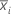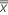# Methods and formulas for Zone Chart

Select the method or formula of your choice.

## Method

The zone chart classifies observations or subgroup means according to their distance from the center line. For each observation or subgroup mean, the corresponding plot point is derived as follows.

1. Each observation or subgroup mean is assigned a weight.
The zone chart consists of four zones that have different weights:
• Zone 1 is between the center line and 1σ and has a weight of 0
• Zone 2 is between 1σ and 2σ and has a weight of 2
• Zone 3 is between 2σ and 3σ and has a weight of 4
• Zone 4 is beyond 3σ and has a weight of 8
2. Each observation or subgroup is then assigned a zone score, which is the value that is actually plotted:
• The first zone score is for the first observation or subgroup mean.
• For subsequent points, zone scores are summed sequentially. Each time a new point crosses the center line, the zone score is reset to zero. If the zone score is greater than the weight assigned to that zone, the process is declared out-of-control at that point.

You can specify weights other than 0, 2, 4, and 8 for the zone scores. You can also choose to reset the cumulative score after each signal.

## Plotted points

First plotted point

Zone score or weight of.

Subsequent plotted point

Sum of sequential weights. Each time a new point crosses the center line, the sum is reset to zero.

## Center line

The center line represents the process average, μ. For a zone chart, μ is the overall average of the individual observations or subgroup averages when the subgroup size is constant.

The default zone chart usesfor μ, the pooled standard deviation for σ when subgroup size > 1, and the average moving range for σ when subgroup size = 1.

By using this site you agree to the use of cookies for analytics and personalized content.  Read our policy